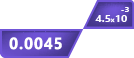# Sig Fig Calculator

Click To Copy

Click To Copy

Click To Copy

Click To Copy

## Other Calculators

Significant figures or sig figs calculator is used to count the number of essential digits in a number. The result contains:

1. Sig figs
2. Number of significant figures
3. Number of decimals
4. E-notation
5. Scientific notation

You can see which digits are significant figures and how many actually are there (no. of decimals). And further you can copy the results.

It can round off the values up to 10 places. On setting up the rounding value, the sig fig counter will round your entered number then tell you how many and which ones are significant values.

## How to use sig fig calculator?

1. Enter a decimal number or an exponential number  in the input box
2. Adjust the round value scale (0 to 10)
3. Hit the calculate button

## What are significant figures?

As the name suggests, it is the number of digits, in a value, that play a significant role in determining its quantity.

These are the figures which are reliable and contribute to a value’s, usually a measurement, accuracy.

So does that mean there are any insignificant figures in values as well?

Yes! There ARE characters in numbers that are not required when using them for measurements or any other purpose. Such numbers are called insignificant figures.

## Sig Figs rules:

Significant figures are determined by a set of rules. These rules mainly focus on the digit zero (0). These rules can be divided into two main headings.

### All digits except zero:

All the digits from 1 to 9 are significant regardless of their position in the value. For example; 2345 has 4 significant figures.

### The digit zero:

Zeros come at three places in a number; start, in between, and end. Each of these zeros has a separate significance.

• #### Trailing zeros

The zeros that come at the end of a number for example 2300 or 23.40.

1. If the trailing zero is after a decimal point then it is significant. Like in the case of 23.40 and 4.00.
2. But if the value is a whole number and does not contain a decimal point then the trailing zeros are insignificant. For example, 2300 has only two significant digits.
3. If the value ends in a zero but there is a decimal point at the end, then th trailing zeros are considered significant. E.g the zeros in ‘100.’ are significant.
• #### Zeros in between

This rule is simple. Any zero which is bracketed by non-zero digits is considered significant. Like the zeros in 2009 and 10.4

• #### Preceding zeros

Preceding zeros are the zeros that are present in a number before any non-zero digit. If;

1. The zero is to represent the value less than zero like in 0.9 then the zero is insignificant.
2. It is a space holder zero, zero after a decimal point and before a sig fig, as the 2nd and 3rd zeros in 0.008 then still it is insignificant.

## Identifying sig figs:

#### Example:

How many significant figures are there in 23.090?

Solution:

1. Digits 2 and 3 are non-zeros hence significant.
2. The 0 after the decimal point is an ‘in-between zero’ so it is also significant
3. 9 is significant for the same reason as 2 and 3.
4. The last 0 is the ‘trailing zero after decimal point’ therefore also significant.

This means there are a total of 5 significant figures in 23.090.

## Rounding off:

Rounding significant figures is done after the mathematical operations are performed e.g adding and subtracting sig figs. To what value you should round off, is a debate for another time. Here you will learn how to round off.

Let’s say your value contains 3 sig figs and you want to round it off to 2 places. Then;

1. If the last number is 5 or greater than 5, you will have to add one to the second place and drop the last digit. E.g 239 rounded off to 2 places is 24.
2. But if the last number is less than 5 then you simply drop it. E.g 452 as 45.

Example:

How many sig figs are there if you round 64013 to 3 places.

Solution:

Rounding off 64013 to 3 digits gives us 640. Because 3 and 1 are smaller than 5.

As the trailing zero in a whole number is insignificant that means there are only 2 significant figures in 640 i.e 6 and 4.

The significant digits calculator also can round off any entered value.